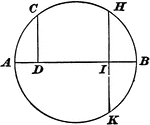### Circle With a Perpendicular Drawn to the Diameter

"If from any point on the circumference of a circle, a perpendicular be let fall upon a given diameter,…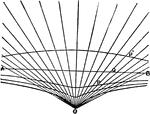### Conchoid

Illustration showing a conchoid, "a curve, shell-like in flexure (whence the name), invented by Nicomedes…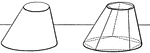### Frustum of Cone to Find Volume

"The volume of a frustum of a circular cone is equivalent to the sum of the volumes of three cones whose…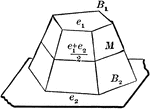### Volume of Frustum of a Pyramid

Diagram used to prove the theorem: "The volume of the frustum of a pyramid (cone) is equal to the sum…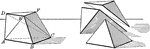### Triangular Pyramid Frustum

The frustum of a triangular pyramid is equivalent to the sum of three pyramids whose common altitude…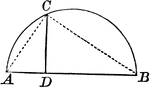### Right Triangle Inscribed in Semicircle Shows Mean Proportional

Right triangle inscribed in semicircle. Illustration shows that the perpendicular from any point in…